Next: Local densities Up: hfbrad23w Previous: Introduction

# The Skyrme Hartree-Fock-Bogolyubov equations

The HFB approximation is based on the use of a trial variational wave function which is assumed to be an independent quasiparticle state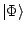. This state, which mixes different eigenstates of the particle number operator, is a linear combination of independent particle states representing various possibilities of occupying pairs of single particle states. Following the notations and phase convention of  we define the particle and pairing density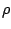and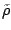matrices by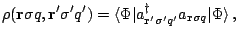(1)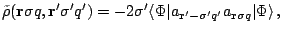(2)

where the operators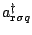and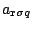create and annihilate a nucleon at the point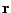having spin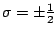and isospin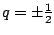. The symmetry properties ofandas well as the relation betweenand the pairing tensor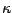(defined for example in ) are discussed in .

The variation of the energy expectation value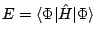with respect toandunder the constraints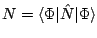and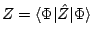(for neutrons and protons) leads to the Hartree-Fock-Bogolyubov equation which reads in coordinate representation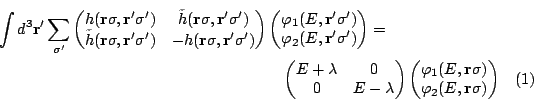where the particle and pairing fields are given by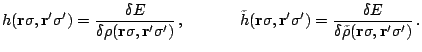(3)

Once again we refer the reader to the article  for the discussion concerning the quasiparticle spectrum, its symmetries and the relations between the components of the HFB spinors and the densities.

SubsectionsNext: Local densities Up: hfbrad23w Previous: Introduction
Jacek Dobaczewski 2005-01-23